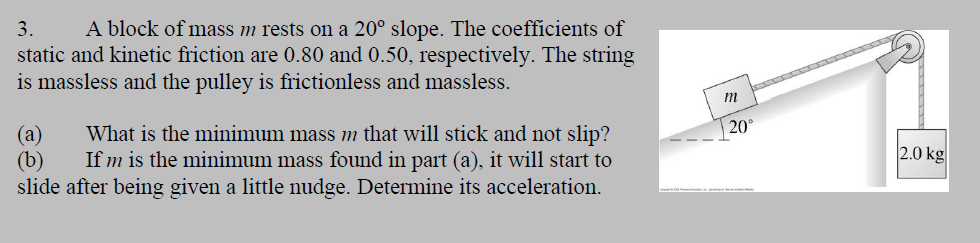#### (Solved): A block of mass m rests on a 20 degree slope. The coefficients of static and kinetic friction are 0. ...

A block of mass m rests on a 20 degree slope. The coefficients of static and kinetic friction are 0.80 and 0.50, respectively. The string is massless and the pulley is frictionless and massless.

(a) What is the minimum mass m that will stick and not slip?

(b) If m is the minimum mass found in part (a), it will start to slide after being given a little nudge. Determine its acceleration.3. A block of mass m rests on a 20° slope. The coefficients of static and kinetic friction are 0.80 and 0.50, respectively. The string is massless and the pulley is frictionless and massless. 20 2.0 kg (a) What is the minimum mass m that will stick and not slip? (b) If m is the minimum mass found in part (a), it will start to slide after being given a little nudge. Determine its acceleration.